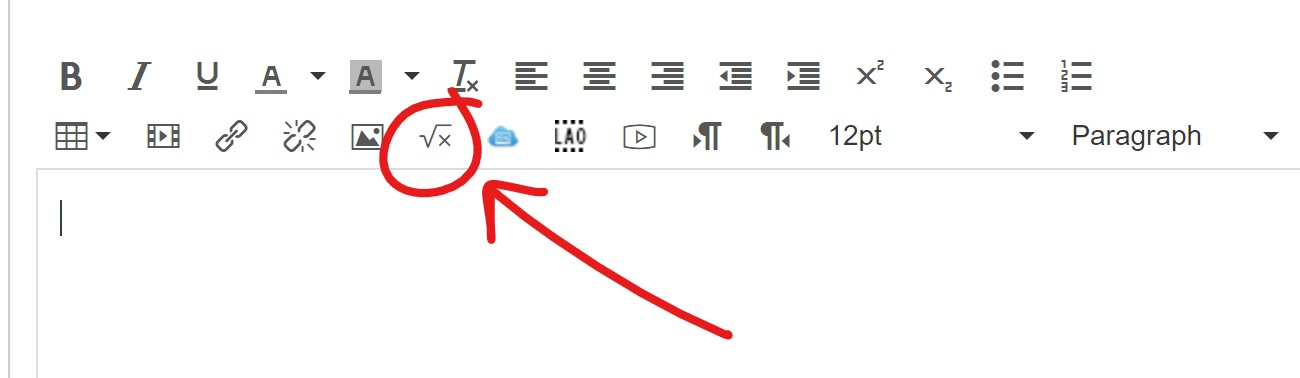# 0.4 - Canvas' Equation Editor

0.4 - Canvas' Equation Editor

On Canvas discussion boards and essay quiz questions you have the option to "Insert Math Equation"When you click on this button you have the option of switching between "Basic" and "Advanced" views. Most of what you will need to do in STAT 200 can be accomplished under the basic view, with the exception of $$\widehat{p}$$. The video below will walk you through a few examples.

## Canvas Equation Editor

The table below contains some of the symbols and functions that you will use most often in this course. Note that \widehat will only work in the advanced view.

 Symbol Code $$\overline x$$ \overline x $$\widehat p$$ \widehat p $$\sum$$ \sum $$\mu$$ \mu $$H_0:$$ H_0 \$: $$s^2$$ s^2

  Link ↥ Has Tooltip/Popover Toggleable Visibility